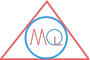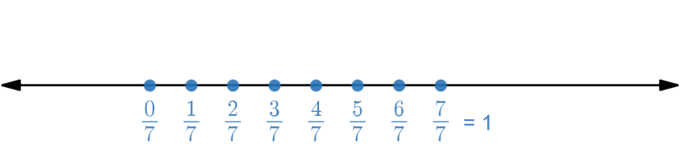MATHS QUERY

# Fraction and its Various Types

## Definition and example of fraction

A fraction is a number which represents a part of a whole.

Fractions in Maths helps us to measure how many parts are there and the size of each part of an object.

To understand fractions, let’s take a real life example of a pizza bought from market and share with 8 friends equally.

The pizza is going to be divided into eight equal pieces assuming that everyone will share one equal piece.

Next, it is time to dive into its mathematics part and see how it can be linked to fractions.

So, a pizza has been cut into total equal pieces of 8 and one friend eats one piece of it.

We can write the one piece of pizza in fractions as $$\frac{1}{8}$$, where, 1 in numerator denote one piece and 8 in denominator denotes total number of pieces.

In fractions we can say one friend is sharing $$\frac{1}{8}th$$ (read as one eighth) part of pizza.

As, everybody share equal piece of pizza, so we can also say everyone has shared $$\frac{1}{8}$$ fraction of pizza.

What we have learnt about fractions from above is that always a fraction has a numerator and a denominator. A denominator keeps the value of total number of parts and numerator holds the value of a part of an whole object.

Hence, we can write in fractions a person has shared $$\frac{1}{8}$$ fraction of a whole pizza.

Lets’ make an interesting twist in pizza sharing and see how fractions are written.

How the fraction is written if a person is absent out of 8, eventually any one friend will get an opportunity to have 2 pieces of it. So finally we have 7 friends and 8 cut pieces of pizza.

That friend who will get 2 pieces, in fractions we can say he shared $$\frac{2}{8}$$ fraction of pizza. Again, denominator has total number of pieces i.e. 8, but numerator value is 2 than 1 now because this friend took 2 pieces.

The rest 6 friends shared 1 piece, so their fraction of pizza shared still remains as $$\frac{1}{8}$$.

Let’s see next some examples of fractions.

$$\frac{5}{11}$$

This is read as five – elevenths.

$$\frac{3}{8}$$ means 3 parts are taken from equally divided 8 parts of whole.

Fractions are always positive, that means numerator and denominator are positive integers.

## What are various types of fraction?

### Unit fraction

A fraction whose numerator is one and denominator can be any positive integer is called a Unit fraction.

$$\frac{1}{4}$$, $$\frac{1}{7}$$, $$\frac{1}{61}$$ etc.

In $$\frac{1}{4}$$, numerator is 1 and denominator 4 which is a positive integer.

### Like fractions

The fractions which have same denominator are called Like fractions.

$$\frac{1}{9}$$, $$\frac{2}{9}$$, $$\frac{7}{9}$$ etc.

So, the above fractions $$\frac{1}{9}$$, $$\frac{2}{9}$$ and $$\frac{7}{9}$$ have the same denominator i.e. 9, so they are like fractions.

### Unlike fractions

The fractions which have different denominator are called Unlike fractions.

$$\frac{7}{9}$$, $$\frac{1}{4}$$, $$\frac{6}{11}$$, $$\frac{5}{8}$$ etc.

So, the above fractions $$\frac{7}{9}$$, $$\frac{1}{4}$$, $$\frac{6}{11}$$ and $$\frac{5}{8}$$ have different denominators i.e. 9, 4, 11 and 8 respectively, so they are Unlike fractions.

### Equivalent fractions

The two or more than two fractions are said to be equivalent when multiplying the numerator and denominator by a same non zero positive number results to the original fraction.

Let’s take an example of $$\frac{1}{3}$$ and $$\frac{3}{9}$$.

Multiply both numerator and denominator by 1:

$$\frac{1}{3} \times \frac{1}{1} = \frac{1}{3}$$

Multiply both numerator and denominator by 2:

$$\frac{1}{3} \times \frac{2}{2} = \frac{2}{6}$$

Multiply both numerator and denominator by 3:

$$\frac{1}{3} \times \frac{3}{3} = \frac{3}{9}$$

Here $$\frac{1}{3}$$ becomes equal to $$\frac{3}{9}$$ when multiplied by $$3$$.
Hence, $$\frac{1}{3}$$ and $$\frac{3}{9}$$ are equivalent fractions.

### Simple fraction

A fraction whose both terms are integers is called a simple fraction.

$$\frac{4}{7}$$, $$\frac{3}{5}$$ etc.

### Complex fraction

A fraction whose one or both terms are fractional numbers is called complex fraction

$$\frac{2}{\frac{5}{7}}$$, $$\frac{7}{\frac{5}{\frac{6}{7}}}$$ etc.

### Decimal fraction

Fractions whose denominators are 10, 100, 1000 etc. are decimal fractions.

$$\frac{3}{10}$$, $$\frac{11}{1000}$$ etc.

### Vulgar fraction

Fractions whose denominators are not 10, 100, 1000 etc. are called vulgar fractions.

$$\frac{1}{5}$$, $$\frac{7}{15}$$ etc.

### Proper fraction

A fraction whose numerator is positive and also less than its denominator is called proper fraction.

$$\frac{7}{12}$$, $$\frac{19}{21}$$ etc.

### Improper fraction

A fraction whose numerator is greater than its denominator is called improper fraction.

$$\frac{11}{5}$$, $$\frac{85}{21}$$ etc.

### Mixed fraction

A fraction which is expressed as combination of integer and a proper fraction is called mixed fraction.

$$2\frac{3}{5}$$here 2 represents integer part and $$\frac{3}{5}$$ represents proper fraction.

### Q) What is fraction?

Fraction is a number which expresses a part of whole. The whole may be a single or group of objects.

### Q) What are types of fraction?

Different types of fraction are proper fraction, improper fraction, mixed fraction, simple fraction, complex fraction, vulgar fraction, unit fraction, like fractions, unlike fractions, decimal fraction and equivalent fractions.

### Q) Can fraction be negative?

No, fraction can't be negative. Fractions are always positive i.e. both numerator and denominator are positive integers.

### Q) What are fundamental operations on fractions?

We can do basic operations of arithmetic on fractions i.e. addition, subtraction, multiplication and division, which are fundamental operations on fractions.

## Solved Examples

### 1) Represent $$\frac{2}{7}$$ on number line.

To represent $$\frac{2}{7}$$ on number line, we divide 0 to 1 into 7 equal parts.### 2) Reduce $$\frac{12}{52}$$ into the lowest term.

$$\frac{12}{52}$$ = $$\frac{12 \div 4}{52 \div 4}$$ = $$\frac{3}{13}$$

Here, HCF of 12 and 52 is 4. So, we divide numerator and denominator by 4.

### 3) What is fraction of 9 hours in a day?

One day has 24 hours. Therefore, we can say that total number of parts are 24 and the value of part of an whole object is 9.

∴ This fraction can be written as:

$$\frac{9}{24}$$ = $$\frac{9 \div 3}{24 \div 3}$$ = $$\frac{3}{8}$$

### 4) What is fraction of prime numbers from 1 to 20?

Prime numbers from 1 to 20 are 2, 3, 5, 7, 11, 13, 17 and 19, which are total 8 in numbers.

We can write the fraction of prime numbers from 1 to 20 as:

$$\frac{8}{20}$$ = $$\frac{8 \div 4}{20 \div 4}$$ = $$\frac{2}{5}$$

### 5) Convert $$5\frac{2}{7}$$ into improper fraction.

$$5\frac{2}{7}$$ = $$5 + \frac{2}{7}$$ = $$\frac{7 \times 5 + 2}{7}$$

= $$\frac{35 + 2}{7}$$ = $$\frac{37}{7}$$

### 6) Which fraction is greater, $$\frac{5}{9}$$ or $$\frac{4}{5}$$?

$$\frac{5 \times 5}{9 \times 5}$$ = $$\frac{25}{45}$$

$$\frac{4}{5} \times \frac{9}{9}$$ = $$\frac{36}{45}$$

$$\frac{36}{45}$$ is greater than $$\frac{25}{45}$$

i.e. $$\frac{4}{5} \gt \frac{5}{9}$$

## Multiple Choice Questions

### 1) The correct form of fraction for the statement 5 out of 9 is

a) $$\frac{9}{5}$$

b) $$\frac{5}{9}$$

c) $$9^5$$

d) $$5^9$$

a) 9

b) 18

c) -18

d) -2

### 3) $$\frac{4}{5}$$ and $$\frac{6}{5}$$ fractions are an example of

a) Like fractions

b) Unlike fractions

c) Mixed fractions

d) Equivalent fractions

### 4) Solve the fraction $$2\frac{4}{3}$$

a) $$\frac{10}{3}$$

b) $$\frac{14}{3}$$

c) $$\frac{11}{3}$$

d) $$\frac{24}{3}$$

### 5) Which fraction is smaller $$\frac{2}{3}$$ or $$\frac{3}{4}$$

a) $$\frac{2}{3}$$

b) $$\frac{3}{4}$$

c) Both are equal

d) None of these

### 6) The lowest term of $$\frac{35}{42}$$is

a) $$\frac{5}{6}$$

b) $$\frac{5}{42}$$

c) $$\frac{35}{21}$$

d) $$\frac{35}{42}$$

### 7) Choose the correct equivalent fraction

a) $$\frac{5}{4} = \frac{4}{5}$$

b) $$\frac{3}{5} = \frac{2}{3}$$

c) $$\frac{7}{2} = \frac{2}{5}$$

d) $$\frac{3}{4} = \frac{15}{20}$$

### 8) Arrange $$\frac{5}{7}$$, $$\frac{3}{8}$$, $$\frac{7}{2}$$, $$\frac{3}{4}$$ in ascending order.

a) $$\frac{3}{8}$$, $$\frac{5}{7}$$, $$\frac{3}{4}$$, $$\frac{7}{2}$$

b) $$\frac{3}{8}$$, $$\frac{7}{2}$$, $$\frac{3}{4}$$, $$\frac{5}{7}$$

c) $$\frac{3}{4}$$, $$\frac{3}{8}$$, $$\frac{5}{7}$$, $$\frac{7}{2}$$

d) $$\frac{5}{7}$$, $$\frac{7}{2}$$, $$\frac{3}{8}$$, $$\frac{3}{4}$$

a) 4

b) 3

c) 19

d) 5

a) 2

b) 7

c) 18

d) 63